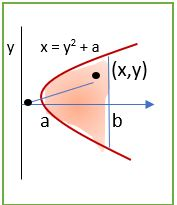# Find the average square distance f from the original to a point (x, y) in the domain D. Assume...

## Question:

Find the average square distance f from the original to a point (x, y) in the domain D. Assume that a = 1 and b = 4. Round the answer to three decimal places.## Double Integral

This problem is based on the application of definite integrals and double integrals. To solve this problem we need to be familiar with the concept of finding the area using integrals. From the given constraints we will define the limits of integration followed by the calculation of the integrals.

We have,

{eq}\displaystyle f(x,y)=x^2+y^2 {/eq}

{eq}\displaystyle a=1\text{ and }b=4 {/eq}

Now,

{eq}\displaystyle x=y^2+1 {/eq}

{eq}\displaystyle 4=y^2+1 {/eq}

{eq}\displaystyle y=\pm \sqrt{3} {/eq}

{eq}\displaystyle x=4 {/eq}

So,

{eq}\displaystyle A=\int_{-\sqrt 3}^{\sqrt 3}(4-y^2-1)dy {/eq}

{eq}\displaystyle A=\left ( 3y-\frac{y^3}{3} \right )_{-\sqrt 3}^{\sqrt 3} {/eq}

{eq}\displaystyle A=4\sqrt 3 {/eq}

Let us now calculate the average square distance,

{eq}\displaystyle f_{avg}=\frac{1}{A}\int \int f(x,y)dA {/eq}

{eq}\displaystyle f_{avg}=\frac{1}{4\sqrt 3}\int_{-\sqrt 3}^{\sqrt 3}\int_{y^2+1}^{4}(x^2+y^2)dxdy {/eq}

{eq}\displaystyle f_{avg}=\frac{1}{4\sqrt 3}\int_{-\sqrt 3}^{\sqrt 3}\left ( \frac{x^3}{3}+y^2x \right )_{y^2+1}^{4}dy {/eq}

{eq}\displaystyle f_{avg}=\frac{1}{4\sqrt 3}\int_{-\sqrt 3}^{\sqrt 3}\left ( \frac{64}{3}+4y^2-\frac{(y^2+1)^3}{3}-y^2(y^2+1) \right )dy {/eq}

Expanding the bracket and applying the limits of integration, we get,

{eq}\displaystyle f_{avg}=\frac{1}{4\sqrt 3}\times \left ( 6\sqrt 3+\frac{1058\sqrt 3}{35} \right ) {/eq}

{eq}\displaystyle f_{avg}=\left ( \frac{6}{4}+\frac{264.5}{35} \right ) {/eq}

{eq}\displaystyle f_{avg}=\left ( \frac{3}{2}+\frac{264.5}{35} \right ) {/eq}

Thus,

{eq}\displaystyle \boxed{\displaystyle f_{avg}=9.057} {/eq}